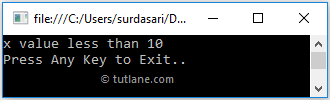# Visual Basic If-Else-If Statement

In Visual Basic, the If-Else-If statement or condition is useful for defining the multiple conditions and executing only the matched condition based on our requirements.

Generally, Visual Basic, if statement or if-else statement is useful when we have one condition to validate and execute the required block of statements. If we have multiple conditions to validate and execute only one block of code, then the If-Else-If statement is useful in our application.

## Syntax of Visual Basic If-Else-If Statement

Following is the syntax of defining the If Else If statement in Visual Basic programming language.

If condition_1 Then
// Statements to Execute if condition_1 is True
ElseIf condition_2 Then
// Statements to Execute if condition_2 is True
ElseIf condition_2 Then
// Statements to Execute if condition_3 is True
....
....
Else
// Statements to Execute if all conditions are False
End If

If you observe the above Visual Basic If-Else-If statement syntax, we defined multiple conditions to execute required statements.

Here, the execution of the If-Else-If statement will start from the top to bottom, and as soon as the condition returns true, the code inside of the If or ElseIf block will be executed, and the control will come out of the loop. If none of the conditions return true, then the code inside of Else block will be executed.

Following is a simple example of using the If-Else-If statement in Visual Basic programming language.

Dim x As Integer = 5
If x = 10 Then
Console.WriteLine("x value equals to 10")
ElseIf x > 10 Then
Console.WriteLine("x value greater than 10")
Else
Console.WriteLine("x value less than 10")
End If

If you observe the above example, the if-else-if statement will start the execution from top to bottom and check if any condition matches or not to execute the respective code block. If no condition matches, the else block will be executed.

## Visual Basic If-Else-If Statement Flow Chart

Following is the flow chart diagram, representing the process flow of the If-Else-If statement in Visual Basic programming language.If you observe the above Visual Basic If-Else-If statement flow chart, when the defined condition is true, the statements within the If condition will execute otherwise, it will move to another condition (Else-If) to check whether the condition is true or not. If no conditions match, then the Else block will be executed.

## Visual Basic If-Else-If Statement Example

Following is the example of defining the If-Else-If statement in Visual Basic programming language to execute the block of code or statements based on the Boolean expression.

Module Module1
Sub Main()
Dim x As Integer = 5
If x = 10 Then
Console.WriteLine("x value equals to 10")
ElseIf x > 10 Then
Console.WriteLine("x value greater than 10")
Else
Console.WriteLine("x value less than 10")
End If
Console.WriteLine("Press Enter Key to Exit..")
End Sub
End Module

The above example shows that we defined the If-Else-If conditions to execute the statements based on defined condition status.

When we execute the above Visual Basic program, we will get the result as shown below.If you observe the above result, all the defined conditions are failing due to that it executed the Else block of statements and printed the required statement in the console window.

This is how we can use the If-Else-If statement in Visual Basic programming language to execute the block of code or statements based on our requirements.